In this article, we will share MP Board Class 10th Maths Book Solutions Pair of Linear Equations in Two Variables Ex 3.5 Pdf, These solutions are solved subject experts from the latest edition books.

## MP Board Class 10th Maths Solutions Chapter 3 Pair of Linear Equations in Two Variables Ex 3.5

Question 1.
Which of the following pairs of linear equations has unique solution, no solution, or infinitely many solutions. In case there is a unique solution, find it by using cross multiplication method.
(i) x – 3y – 3 = 0; 3x – 9y – 2 = 0
(ii) 2x + y = 5; 3x + 2y = 8
(iii) 3x – 5y = 20; 6x -10y = 40
(iv) x – 3y – 7 = 0; 3x – 3y – 15 = 0
Solution:
(i) For x – 3y – 3 = 0, 3x – 9y – 2 = 0
∴ a1 = 1, b1 = – 3, C1 = – 3, a2 = 3, b2 = – 9, C2 = -2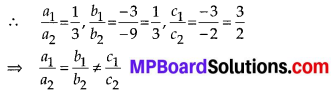∴ The given system has no solution.

(ii) 2x + y – 5 = 0, 3x + 2y – 8 = 0
∴ a1 = 2, b1 = 1, c1 = -5, a2 = 3, b2 = 2, c2 = -8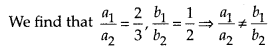∴ The given system has a unique solution.
Now, using cross multiplication method, we have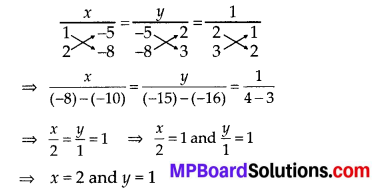(iii) For 3x – 5y – 20 = 0, 6x – 10y – 40 = 0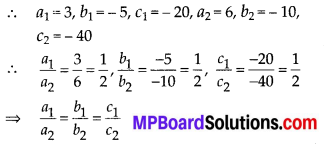∴ The given system of linear equation has infinitely many solutions

(iv) For x – 3y – 7 = 0, 3x – 3y – 15 = 0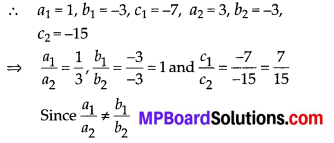∴ The given statement has unique solution. Now, using cross multiplication method, we have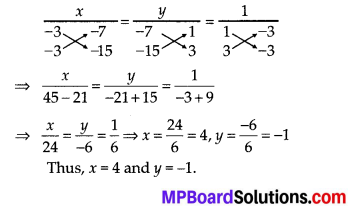Question 2.
(i) For which values of a and b does the following pair of linear equations have an infinite number of solutions ?
2x + 3y = 7; (a – b) x + (a + b) y = 3a + b – 2
(ii) For which value of k will the following pair of linear equations have no solution ?
3x + y = 1; (2k – 1) x + (k – 1) y = 2k + 1
Solution:
(i) We have, 2x + 3y = 7 and
(a – b) x + (a + b) y = (3a + b – 2)
⇒ 2x + 3y – 7 = 0
and (a – b) x + (a + b) y -(3a + b – 2) = 0
a1 = 2, b1 = 3, c1 = – 7, a2 = (a- b) , b2 = (a + b) , c2 = -(3a + b – 2)
For infinite number of solutions,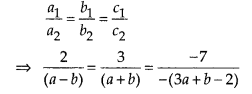From the first two equations, we get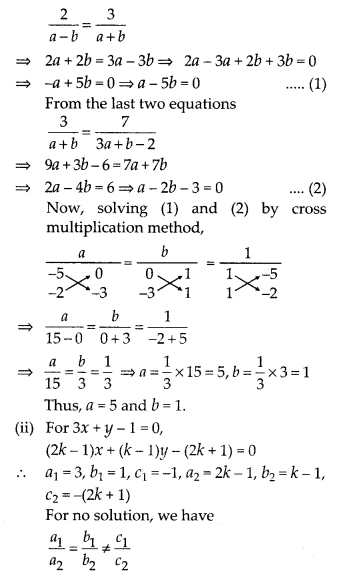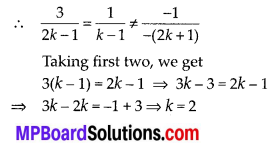Question 3.
Solve the following pair of linear equations by the substitution and cross-multiplication methods: 8x + 5y = 9; 3x + 2y = 4
Solution:
Method-1 [Substitution method]:
8x + 5y = 9 … (1)
3x + 2y = 4 … (2)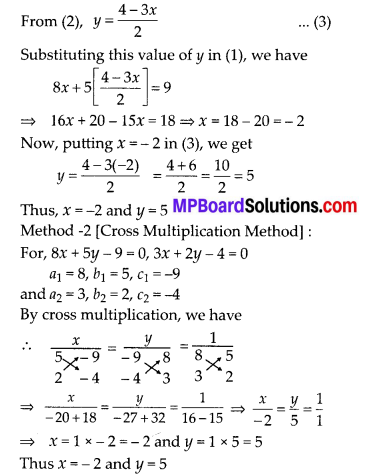Question 4.
Form the pair of linear equations in the following problems and find their solutions (if they exist)by any algebraic method:
(i) A part of monthly hostel charges is fixed and the remaining depends on the number of days one has taken food in the mess. When a student A takes food for 20 days she has to pay ₹ 1000 as hostel charges whereas a student B, who takes food for 26 days, pays ₹ 1180 as hostel charges. Find the fixed charges and the cost of food per day.
(ii) A fraction becomes 1/3 when 1 is subtracted from the numerator and it becomes 1/4 when 8 is added to its denominator. Find the fraction.
(iii) Yash scored 40 marks in a test, getting 3 marks for each right answer and losing 1 mark for each wrong answer. Had 4 marks been awarded for each correct answer and 2 marks been deducted for each incorrect answer, then Yash would have scored 50 marks. How many questions were there in the test?
(iv) Places A and B are 100 km apart on a highway. One car starts from A and another from B at the same time. If the cars travel in the same direction at different speeds, they meet in 5 hours. If they travel towards each other, they meet in 1 hour. What are the speeds of the two cars?
(v) The area of a rectangle gets reduced by 9 square units, if its length is reduced by 5 units and breadth is increased by 3 units. If we increase the length by 3 units and the breadth by 2 units, the area increases by 67 square units. Find the dimensions of the rectangle.
Solution:
(i) Let the fixed charges = ₹ x
and charges of food per day = ₹ y
For student A : Number of days = 20
∴ Cost of food for 20 days = ₹ 20y
According to the problem,
x + 20y = 1000
⇒ x + 20y – 1000 = 0 …. (1)
For student B : Number of days = 26
Cost of food for 26 days = ₹ 26y
According to the problem,
x + 26y = 1180
⇒ x + 26y – 1180 = 0 … (2)
Solving these by cross multiplication, we get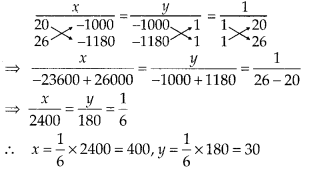Thus x = 400 and y = 30
∴ Fixed charges = ₹ 400
and cost of food per day = ₹ 30(ii) Let the numerator = x
and the denominator = y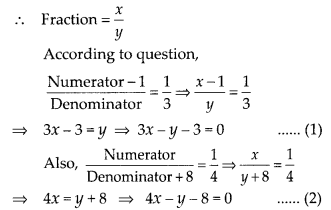From equations (1) and (2) , we have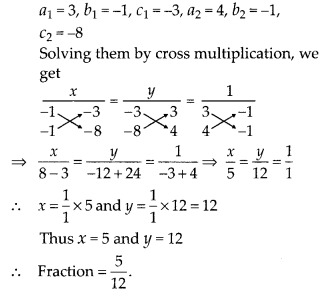(iii) Let the number of correct answers = ₹ x
and the number of wrong answers = ₹ y
Case-I: Marks for all correct answers
= (3 × x) = 3x
Mark for all wrong answers = (1 × y) = y
∴ According to the condition :
3x – y = 40 ⇒ 3x – y – 40 = 0
Case-II: Mark for all correct answers
= (4 × x)=
Marks for all wrong answers = (2 × y)
= 2y
∴ According to the condition :
4x – 2y = 50
⇒ 2x – y = 25 ⇒ 2x – y – 25 = 0 … (2)
From (1)and (2) , we have a1 = 3, b1 = -1, c1 = -40, a2 = 2, b2 = -1, c2 = -25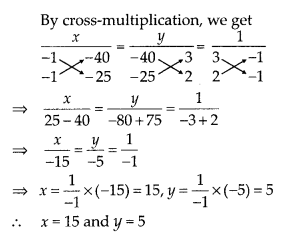Now, total number of questions = [Number of correct answers] + [Number of wrong answers]
= 15 + 5 = 20
Thus, required number of questions = 20.(iv) Let the speed of car-I be x km/hr.
and the speed of car-II be y km/hr.
Case-I: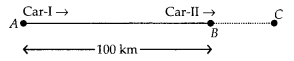Distance travelled by car-I = AC
∵ AC = time × speed = 5 × x km, AC = 5x
Distance travelled by car-II, BC = 5y
Since AB = AC – BC,
100 = 5x – 5y
⇒ 5x – 5y – 100 = 0
⇒ x – y – 20 = 0 …. (1)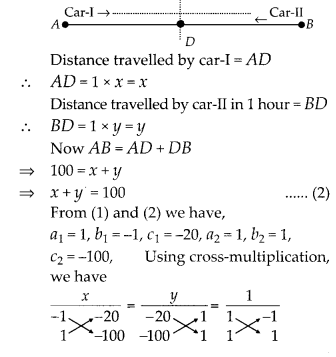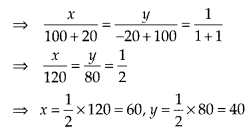Thus, speed of car-I = 60 km/hr
Speed of car-II = 40 km/hr(v) Let the length of the rectangle = x units
and the breadth of the rectangle = y units
∴ Area of rectangle = x × y = xy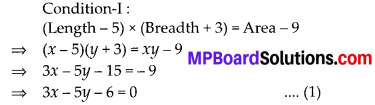Condition-II:
(Length + 3) × (Breadth + 2) = Area + 67
⇒ (x + 3) (y + 2)= xy + 67 ⇒ 2x + 3y + 6 = 67
⇒ 2x + 3y – 61 = 0….(2)
Now, using cross multiplication method in (1)and (2) , where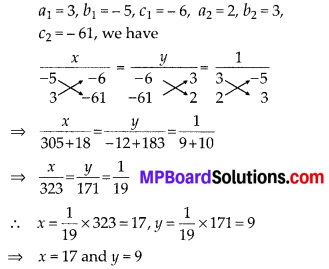Thus, length of the rectangle = 17 units and breadth of the rectangle = 9 units.